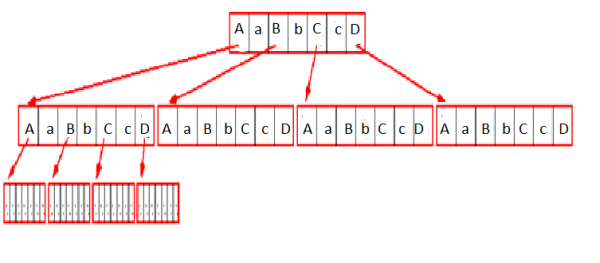# m-ary tree

Data Structure AlgorithmsAnalysis of AlgorithmsAlgorithms

#### Big Data Hadoop

Best Seller

89 Lectures 11.5 hours

#### Practical Data Science using Python

22 Lectures 6 hours

#### Data Science and Data Analysis with Python

50 Lectures 3.5 hours

An m-ary tree in computer science is defined as a collection of nodes normally represented hierarchically in the following manner.

• The tree is started at the root node.
• Each node of the tree maintains a list of pointers to its child nodes.
• The number of child nodes is less than or equal to m.

A typical representation of m-ary tree implements an array of m references (or pointers) to store children (Note that m is an upper bound on number of children).

An m-way search tree

a. is empty or

b. consists of a root containing b (1<=b<m) keys, kb, and a set of sub-trees, Ta, (a = 0..b), such that

• if k is a key in T0, then k <= k1
• if k is a key in Ta (0<a<b), then ka <= k <= ka+1
• if k is a key in Tb, then k > kb and
• all Ta are nonempty m-way search trees or all Ta are emptyImage of m-ary Tree

The height of a complete m-ary tree associated with n nodes is ceiling(logmn).

A B-tree of order m is an m-way tree in which

a. all leaves should be on the same level and

b. all nodes except for the root and the leaves have minimum m/2 children and maximum m children. The root has minimum 2 children and maximum m children.